Smartick is a fun way to learn math!Oct06

# Discover Geometric Figures Found in Our Environment

In today’s post, we’re going to talk about geometric figures that, without us noticing, play a much bigger role in our lives than we think. We’ve looked at different geometric shapes in previous posts, you can see them here.

### How many geometric shapes can you find?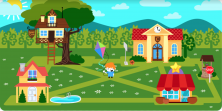Let’s look at some of them:A triangle is a polygon that is formed from 3 points joined by straight lines. The points where the lines intersect are the vertices, and the segments make up the sides.

In this case, we’ve can see an isosceles triangle on the roof of the Smartick school. Isosceles triangles have two equal sides and two equal angles.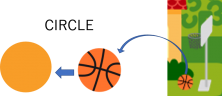A circle is a geometric figure that is made by drawing a line in the shape of a curve, so it is always the same distance from a point that we call the center.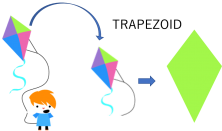A trapezoid is a polygon with four sides, but none of its sides are parallel to each other. Trapezoids can be symmetrical or asymmetrical, depending on their characteristics.

In this case, the kite is a symmetrical trapezoid. These trapezoids have two pairs of equal sides and one of these pairs of sides is smaller than the other.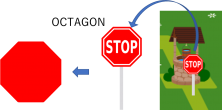An octagon is a polygon with eight sides. A regular octagon is a polygon that has eight equal sides and eight equal angles. The sum of the interior angles of an octagon is always 1080º. Depending on the sizes of their interior angles, octagons can also be arranged into convex octagons, which have obtuse interior angles (bigger than 180º), or irregular octagons, which can have sides and angles that are not equal, as well as both concave and convex angles.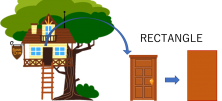A rectangle is a four-sided polygon, with two pairs of equal sides. Its angles are all 90 degrees.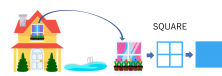A square is a four-sided polygon. All of its sides are equal, and all its angles are 90 degrees.

We’ve found lots of geometric figures!!

I’m sure you can find many more… Sign up for a free trial at Smartick today!

Fun is our brain’s favorite way of learning
Diane Ackerman
Smartick is a fun way to learn math
• 15 fun minutes a day